Introduction to Fixed Income Valuation | IFT World
IFT Notes for Level I CFA® Program
IFT Notes for Level I CFA® Program

# Part 3

## 3. Prices and Yields: Conventions For Quotes and Calculations

### 3.1.     Flat Price, Accrued Interest, and the Full Price

When a bond is between coupon dates, its price has two parts: flat price and accrued interest. The sum of the two parts is called the full price or dirty price.

Full price or dirty price = Flat price + Accrued interestFlat price = Full price – Accrued interest
Accrued interest =where:
t = number of days since the last coupon payment
T = number of days between coupon payments
PMT = interest payment per period

As indicated in the above equation, the accrued interest is based on the number of days since the last coupon payment and the number of days between coupon payments. Here are two conventions for calculating the number of days:

• Actual convention: Uses the actual number of days, including weekends, holidays and leap days as in our example above.
• 30/360 convention: Assumes 30 days in a month and 360 days in a year.

Illustration of Flat Price and Dirty Price through an Example

Assume you own a two-year bond issued on 10th January, 2017 with a par value of $100. The bond pays a coupon of 8% half-yearly. The first coupon is due on 10th July 2017, the next on 10th January 2018, the third on 10th July 2018, and the final one is paid along with principal at maturity date on 10th January 2019. The market discount rate is equal to the coupon rate and so the flat price is 100. An investor wants to purchase the bond from you on 31st May, 2017. What is the full price he must pay? You have held the bond for 140 days (since the bond was issued) and earned an interest for this period even though it has not been paid. The next coupon payment is$4. Assuming each period is 180 days the accrued interest is 4 x (140/180) = 3.11. The full price is 100.00 + 3.11 = 103.11. This is the amount you will receive on selling the bond.

Note that it is the flat (or clean) price which is quoted. The accrued interest increases every day until the coupon payment. So, if the accrued interest was included there would be a variation in bond quotes on a daily basis and a sudden drop after the coupon payment is made, which is misleading.

The full price of a bond can also be calculated using the equation shown below.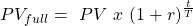where:
t = number of days since the last coupon payment
T = number of days between coupon payments
PV = present value of the bond (not the flat price)

Example

A 5% French corporate bond is priced for settlement on 14 July 2015. The bond makes semi-annual coupon payments on 1 March and 1 September of each year and matures on 1 September 2020. Assuming a 30/360 day count convention for accrued interest, calculate the full price, the accrued interest, and the flat price per EUR 100 of par value for the following three yields-to-maturity: (I) 4.75%, (II) 5.00% and (III) 5.10%.

Solution:

Given the 30/360 day-count convention, there are 134 days between the last coupon on 1 March 2015 and the settlement date on 14 July 2015 (120 days for full months of March, April, May and June plus 14 days in July). Therefore, the fraction of the coupon period that has gone by is assumed to be 134/180. At the beginning of the period, there are 11 periods to maturity.

I) Stated annual YTM of 4.75%

The price at the beginning of the period is 101.198 per 100 of par value.

PV == 101.198
The full price on 14 July is PV(full) =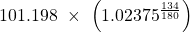= 102.979
The accrued interest is AI == 1.861

The flat price is PV(flat) = 102.979 – 1.861 = 101.118

II) Stated annual YTM of 5.00%

The price at the beginning of the period is 100.00 per 100 of par value.

PV == 100.00
The full price on 14 July is PV(full) =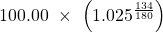= 101.855
The accrued interest is AI == 1.861

The flat price is PV(flat) = 101.855 – 1.861 = 99.9942

III) Stated annual YTM of 5.10%

The price at the beginning of the period is 99.5256 per 100 of par value.

PV =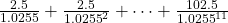= 99.5256
The full price on 14 July is PV(full) == 101.4088
The accrued interest is AI == 1.861

The flat price is PV(flat) = 101.4088 – 1.861 = 99.5478

The flat price of the bond is a little below par value, even though the coupon rate and the yield-to-maturity are equal, because the accrued interest does not take into account the time value of money. The accrued interest is the interest earned by the owner of the bond for the time between the last coupon payment and the settlement date. However, that interest income is not received until the next coupon date. The calculation of accrued interest in practice neglects the time value of money. Therefore, compared to theory, the reported accrued interest is a little “too high” and the flat price is a little “too low.”

### 3.2.     Matrix Pricing

One can easily get information at what price a stock is trading. But, it is not easy to determine the price of most bonds as they do not trade as often as equity. Matrix pricing is an estimation process to find the market price of a not-so-frequently traded bond based on the prices of comparable bonds with similar times-to-maturity, type of issuer, coupon rates, and credit quality. This is also applicable for bonds that have not been issued yet.

For example, consider a three-year, 4% semi-annual bond X that you are trying to value. Comparable bonds whose prices are known are shown in the matrix below. Based on the four bonds, we can determine the price of bond X.

 Matrix Pricing 2% coupon 3% coupon 4% coupon 5% coupon Two years 98.5 102.25 3.786% 3.821% Three years Bond X Four years Five years 90.25 99.125 4.181% 4.196%

There are five steps to determine the value of a bond using the matrix pricing method:

1. Determine the YTM of comparable bonds, which is given to us already. If it were not given, you can calculate the YTM using the price and coupon. For example, YTM of a two-year, 3% coupon is: N = 4; PMT = 1.5; FV = 100; PV = -98.5; CPT I/Y. I/Y = 3.786%.
2. Determine the average yield for two-year bonds: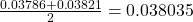3. Determine the average yield for five-year bonds:4. Calculate the three-market discount rate using linear interpolation. We have the data (yield) for two-year and five-year bonds, but not a three-year bond. From the graph you can see that the YTM of a three-year bond = 0.038 + x.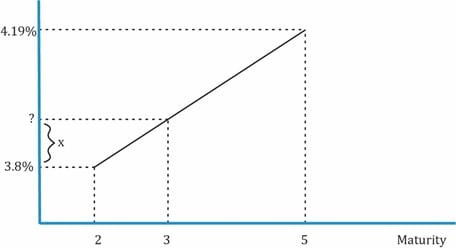x can be calculated as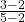x (0.041885 – 0.038035) = 0.001283. Discount rate for the three-year bond =  = 3.39%.

1. Using as the discount rate, compute the price for the three-year bond.

N = 6; I/Y = 1.966; PMT = 2; FV = 100; CPT PV. PV = -100.1906.

A few points to be noted on matrix pricing:

• Matrix pricing is used when underwriting new bonds to estimate the required yield spread over the benchmark rate.
• Benchmark rate is Libor, or a government bond with the same maturity as the bond being priced.
• Assume the YTM for a new bond is calculated using the matrix pricing method as 2.2% and a comparable government bond has a yield of 2.0%. The difference between the yield on a new bond over its benchmark rate is called the required yield spread or spread over the benchmark. In this case, it is 0.2%.
• Yield spreads are always specified in basis points where 1 basis point is one-hundredth of a percentage point. In this case, it is 20 basis points.

Example

An analyst decides to value an illiquid 3-year, 3.75% annual coupon payment corporate bond. Given the following information and using matrix pricing, what is the estimated price of the illiquid bond? Additional information: 2-year, 4.75% annual coupon payment bond priced at 105.60 and 4-year, 3.5% annual coupon payment bond priced at 103.28.

Solution:
The required yield on the 2-year, 4.75% bond priced at 105.60 is 1.871%.
The required yield on the 4-year, 3.50% bond priced at 103.28 is 2.626%.
The estimated market discount rate for a 3-year bond having the same credit quality is the average of two required yields: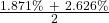= 2.249%.
The estimated price of the illiquid 3-year, 3.75% annual coupon payment corporate bond is 104.3078 per 100 of par value.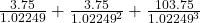= 104.3078

** IFT Website Un-available on Monday 2-October-2023, 0700-1200 GMT **
This is default text for notification bar